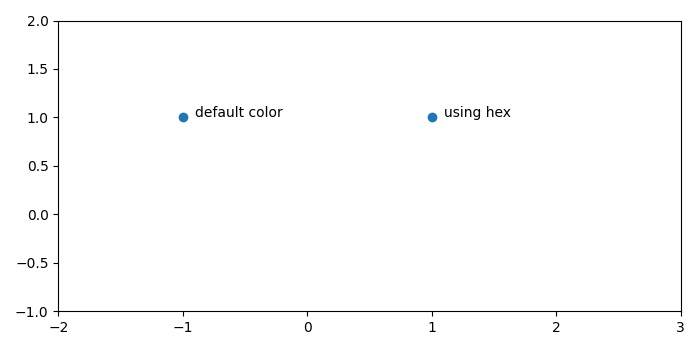# How to get the default blue colour of matplotlib.pyplot.scatter?

MatplotlibPythonData Visualization

#### Python Data Science basics with Numpy, Pandas and Matplotlib

Most Popular

63 Lectures 6 hours

#### Data Visualization using MatPlotLib & Seaborn

11 Lectures 4 hours

#### MatPlotLib with Python

9 Lectures 2.5 hours

The default color of a scatter point is blue. To get the default blue color of matplotlib scatter point, we can annotate them using annotate() method.

## Steps

• Create a figure and a set of subplots using subplots() method.
• Plot a scatter point at (-1, 1) location.
• Add some label for that point.
• Plot a scatter point at (-0.9, 1) location.
• Add some label for that point.
• Plot a scatter point at (1.9, 1) location.
• Add some label for that point.
• Scale the x and y axes using xlim and ylim method.
• To display the figure, use show() method.

## Example

from matplotlib import pyplot as plt
plt.rcParams["figure.figsize"] = [7.00, 3.50]
plt.rcParams["figure.autolayout"] = True
fig, ax = plt.subplots()
ax.scatter(-1, 1)
ax.annotate("default color", xy=(-0.9, 1))
ax.scatter(1, 1, c='#1f77b4')
ax.annotate("using hex", xy=(1.1, 1))
ax.set_xlim(-2, 3)
ax.set_ylim(-1, 2)
plt.show()

## Output